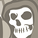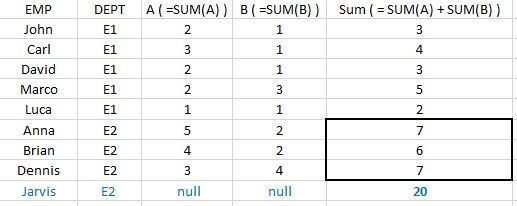# App Development

Discussion board where members can learn more about Qlik Sense App Development and Usage.

Announcements
Welcome to Qlik Community! Check out our new navigation! FIND OUT MORE
cancel
Showing results for
Did you mean:Contributor II

## Displaying department results for certain employees

Hello,

I am trying to display department results only for certain employees. Please see below simplified case.

For employee Jarvis (or those, who do not have A and B values), i wish to display those sums, grouped by his DEPT.How can I do this with set analysis?

Thank you in advance!

Labels (3)

• ### sums

1 Solution

Accepted SolutionsMVP

on frond end, create a straight table

Dimension:

Employee

Dept

Expression:

=if((sum(A)+Sum(B))=0,
sum(total <Dept> aggr(sum(A)+Sum(B),Dept)),sum(A)+Sum(B))

2 RepliesMVP

Would you need script solution or front end?MVP

on frond end, create a straight table

Dimension:

Employee

Dept

Expression:

=if((sum(A)+Sum(B))=0,
sum(total <Dept> aggr(sum(A)+Sum(B),Dept)),sum(A)+Sum(B))Tags
Community Browser